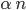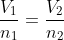×
Get Full Access to Introductory Chemistry - 5 Edition - Chapter 11 - Problem 47p
Get Full Access to Introductory Chemistry - 5 Edition - Chapter 11 - Problem 47p

×

# Solved: A balloon contains 0.128 mol of gas and has a volume of 2.76 L. If an additionalISBN: 9780321910295 34

## Solution for problem 47P Chapter 11

Introductory Chemistry | 5th Edition

• Textbook Solutions
• 2901 Step-by-step solutions solved by professors and subject experts
• Get 24/7 help from StudySoup virtual teaching assistantsIntroductory Chemistry | 5th Edition

4 5 1 283 Reviews
12
5
Problem 47P

A balloon contains 0.128 mol of gas and has a volume of 2.76 L. If an additional 0.073 mol of gas is added to the balloon, what is its final volume?

Step-by-Step Solution:

Step 1 of 2

Here, we need to calculate the final volume of a gas under same condition.

We know,

Avogadro’s law states that the volume of a gas is directly proportional to the amount (in moles).

VThus,Step 2 of 2

## Discover and learn what students are asking

Calculus: Early Transcendental Functions : Exponential and Logarithmic Functions
?In Exercises 7-22, solve for $$x$$. $$4^{x}=64$$

Calculus: Early Transcendental Functions : Introduction to Functions of Several Variables
?In Exercises 7-18, find and simplify the function values. $$g(x, y)=\int_{x}^{y} \frac{1}{t} d t$$ (a) (4,1) (b) (6,3)

Statistics: Informed Decisions Using Data : Properties of the Normal Distribution
?In Problems 29 and 30, draw a normal curve and label the mean and inflection points. ? = 30 and ? = 10

Unlock Textbook Solution

Enter your email below to unlock your verified solution to:

Solved: A balloon contains 0.128 mol of gas and has a volume of 2.76 L. If an additional